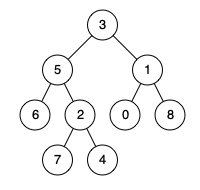# Tree - 236. Lowest Common Ancestor of a Binary Tree

236. Lowest Common Ancestor of a Binary Tree

Given a binary tree, find the lowest common ancestor (LCA) of two given nodes in the tree.

According to the definition of LCA on Wikipedia: “The lowest common ancestor is defined between two nodes p and q as the lowest node in T that has both p and q as descendants (where we allow a node to be a descendant of itself).”

Given the following binary tree:  root = [3,5,1,6,2,0,8,null,null,7,4]Example 1:

``````Input: root = [3,5,1,6,2,0,8,null,null,7,4], p = 5, q = 1
Output: 3
Explanation: The LCA of nodes 5 and 1 is 3.

``````

Example 2:

``````Input: root = [3,5,1,6,2,0,8,null,null,7,4], p = 5, q = 4
Output: 5
Explanation: The LCA of nodes 5 and 4 is 5, since a node can be a descendant of itself according to the LCA definition.
``````

Note:

• All of the nodes' values will be unique.
• p and q are different and both values will exist in the binary tree.

go：

``````/**

* Definition for TreeNode.

* type TreeNode struct {
*     Val int
*     Left *ListNode
*     Right *ListNode
* }
*/
func lowestCommonAncestor(root, p, q *TreeNode) *TreeNode {
if root == nil || root == p || root == q {
return root
}

left := lowestCommonAncestor(root.Left, p, q)
right := lowestCommonAncestor(root.Right, p, q)

if left != nil && right != nil {
return root
}

if left == nil {
return right
} else {
return left
}
}
``````

Nothing just happens, it's all part of a plan.